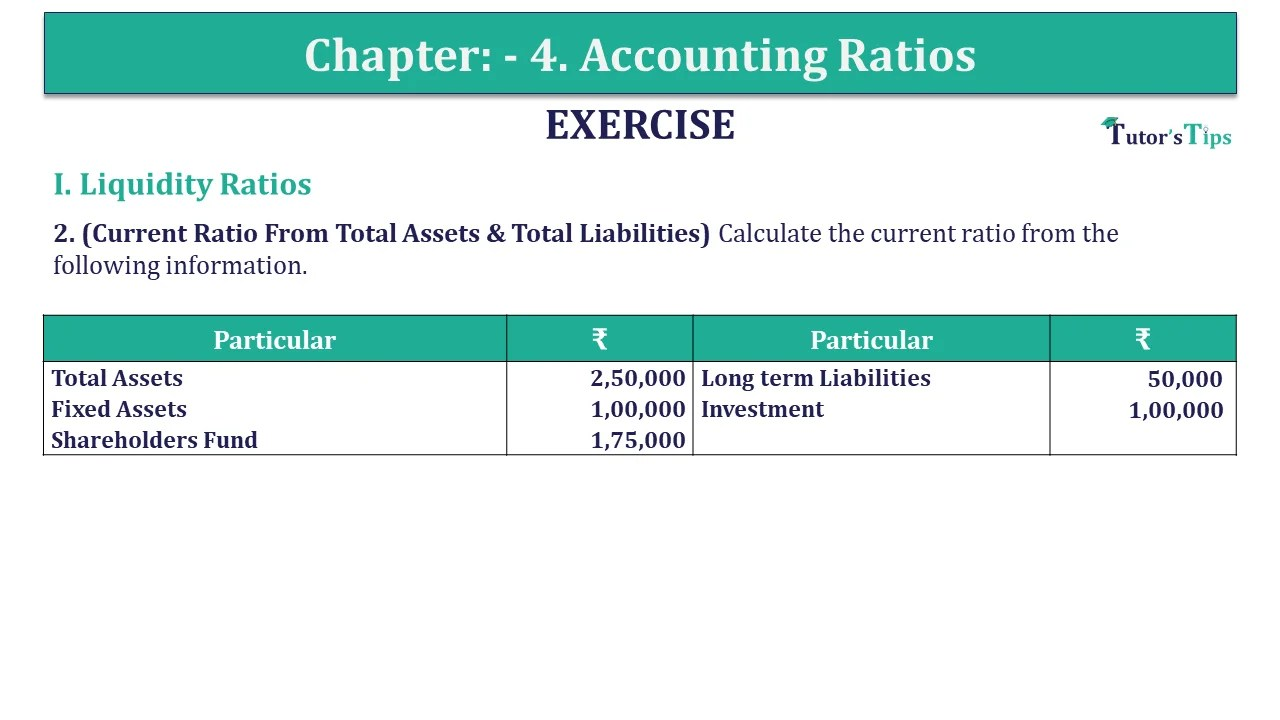# Question 02 Chapter 4 of +2-B – USHA Publication 12 ClassQuestion 02 Chapter 4 of +2-B

I. Liquidity Ratios

2. (Current Ratio From Total Assets & Total Liabilities) Calculate the current ratio from the following information.

 Particular ₹ Particular ₹ Total Assets 2,50,000 Long term Liabilities 50,000 Fixed Assets 1,00,000 Investment 1,00,000 Shareholders Fund 1,75,000

## The solution of Question 02 Chapter 4 of +2-B: –

 Total Assets = Fixed Assets + Investment + Current Assets ₹ 2,50,000 = ₹ 1,00,000 + ₹ 1,00,000 + Current Assets Current Assets = ₹ 50,000

 Total Assets = Total Liabilities (Shareholder’s Fund + Long term Liabilities + Current Liabilities) ₹ 2,50,000 = ₹ 1,75,000 + ₹ 50,000 + Current Liabilities Current Liabilities = ₹ 25,000

 Current Ratio= Current Assets Current Liabilities

 Current Ratio= 50,000 25,000

Current Ratio=2:1

What are Liquidity Ratios – Formulas and Examples